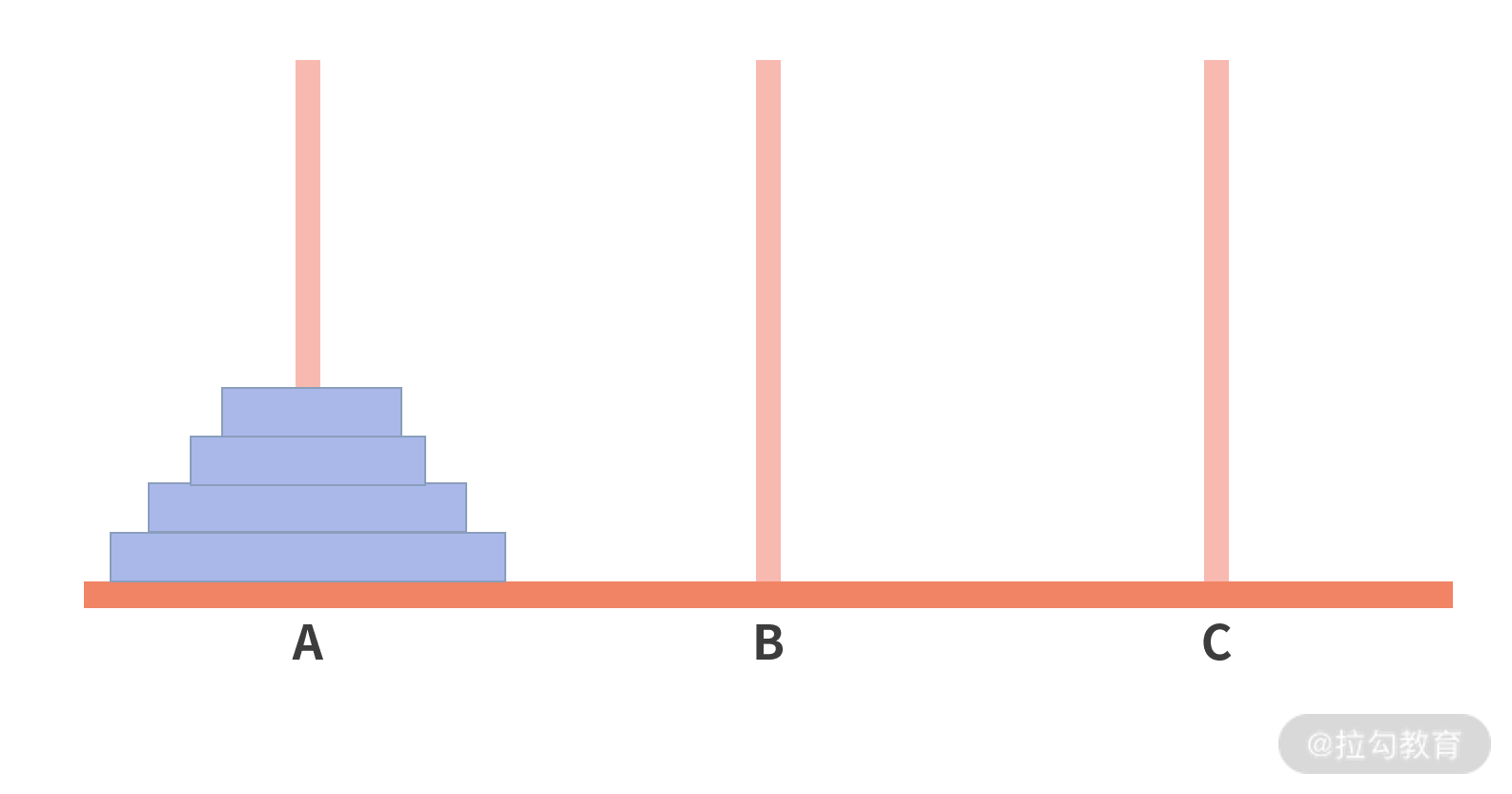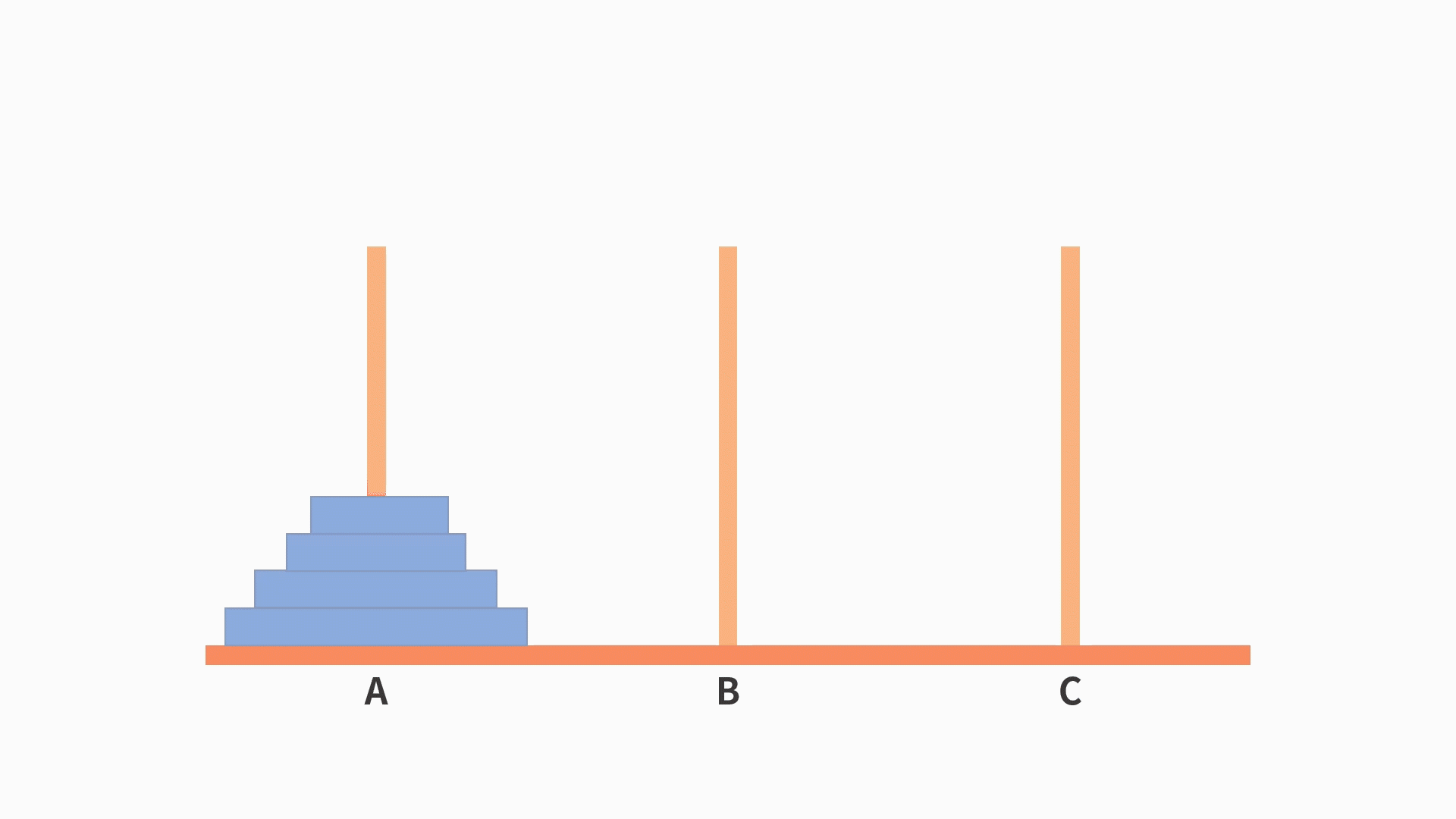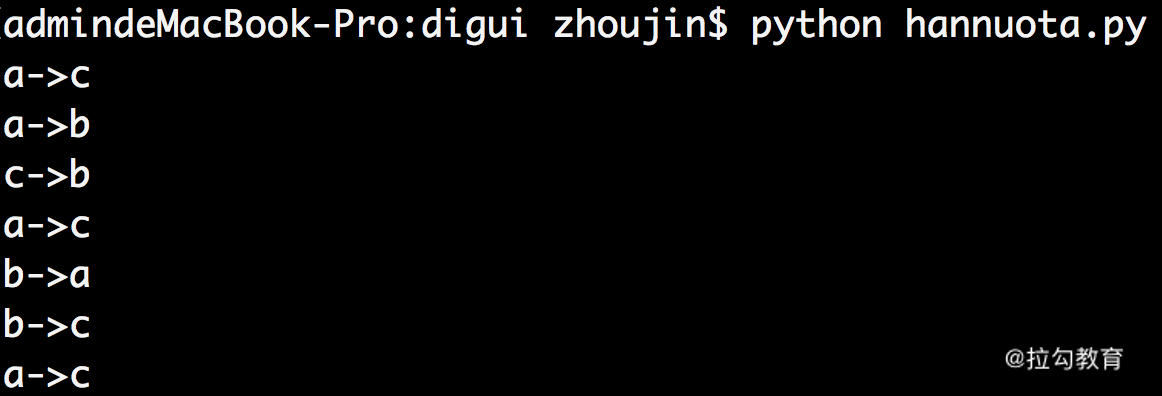15 递归：如何计算汉诺塔问题的移动步数？

汉诺塔问题及其代码实现1.汉诺塔问题解密……

2.汉诺塔问题的代码实现

def hanoi(N,x,y,z):

if N == 1:

print x + '->' + z

else:

hanoi(N - 1, x, z, y)

print x + '->' + z

hanoi(N - 1, y, x, z)

• 首先，函数调用自己，把“合并盘”从 x 移动到 y；
• 然后，把“大盘子”从 x 移动到 z；
• 最后，函数再调用自己，把“合并盘”从 y 移动到 z。

hanoi(3, 'a', 'b', 'c')递归——自己调用自己的程序开发思想

• 第一个问题，递归必须要有终止条件，否则程序就会进入不停调用自己的死循环。

• 第二个问题，写代码之前需要先写出递归公式。 在汉诺塔问题中，递归公式是H(N)=H(N-1)+1+H(N-1)，这也是递归函数代码中除了终止条件以外的部分。

def fun(N,x):

if condition(N):

xxx

else:

fun(N1,x)

• 终止条件的判断基于某个入参 N，如果满足，则函数不再调用自己，终止递归；如果还不满足，则进入到递归体。
• 在递归体中，终止条件判断的入参 N 一定会发生改变。通常而言，是变成比 N 小的一个数值N1。只有这样，递归才能慢慢向终止条件靠近。在递归体中，基于新的参数 N1，再调用函数自身 fun(N1,x)，完成一次递归操作。

递归思维的应用

1.阶乘问题

• 对于终止条件而言，当 n=1 时，返回的值为 1!=1。
• 对于递归体而言，需要先写出递归公式。根据阶乘公式的定义可知，当 n>1 时，H(n)=n!=1×2×...×(n-2)×(n-1)×n= [1×2×...×(n-2)×(n-1)]×n=n×(n-1)!= n×H(n-1)。

def jiecheng(n):

if n == 1:

return 1

else:

return n * jiecheng(n-1)

2.斐波那契序列问题

F(1)=1

F(2)=1

F(3)=1+1=2

F(4)=1+2=3

• 斐波那契数列的终止条件很显然，就是当 n 为 1 或 2 时，返回值就是 1；
• 而它的递归体可以根据斐波那契数列的定义得到，也就是 F(n)=F(n-1)+F(n-2)。

def fib(n):

if n == 1 or n == 2:

return 1

else:

return fib(n-1) + fib(n-2)

• 在第 2 行，判断 n 是否为 1 或 2。
• 如果是，则第 3 行返回 1；
• 反之，则跳转到第 5 行，返回前两项之和，即 fib(n-1)+fib(n-2)。

递归的优缺点

def fib(n):

if n == 1 or n == 2:

return 1

else:

print "fib: " + str(n-1)

print "fib: " + str(n-2)

return fib(n-1) + fib(n-2)

小结——递归与循环

• 循环对于迭代的次数更敏感，绝大多数场景会定义一个用来计数的变量 i，来控制循环的次数；
• 递归对于迭代次数不敏感，取决于什么时候满足终止条件。

• 对于循环而言，每一轮处理的问题难度几乎是一样的；
• 递归则是缩小搜索范围（例如二分查找）的思路，一般而言，每轮处理的问题相对上一轮而言是更简单的。